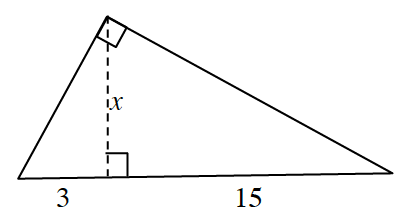### Home > PC3 > Chapter Ch8 > Lesson 8.2.1 > Problem8-62

8-62.

The diagram below has three right triangles. How are these three triangles related? Use this relationship to calculate the value of $x$ in the diagram.These are similar triangles. Set up a proportion and solve for $x$.

$\frac{3}{x}=\frac{x}{15}$